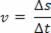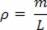Sound and Oscillation

# Taylor’s formula

Taylor’s formula makes it possible to determine the speed of waves on strings that are tensioned, such as those on musical instruments.

The figure below shows the creation of a periodic transverse wave . We give the name of periodic waves to those that are produced by sources that perform periodic oscillations , that is, they are waves that repeat themselves at regular intervals of time.

According to the figure above, in a wave formed by a string, we can identify the crests , which are the highest points of the pulses; the valleys , which are the lowest points of the wave pulse; the amplitude , which corresponds to the greatest distance that each common point of the wave presents (for example, the distance between a crest and a valley); the period , which is the time interval for each homogeneous point of the wave to perform an oscillation; the frequency , which is the number of complete oscillations that each homogeneous point of the wave performs; and finally the wavelength ,which represents the distance between two crests or two valleys.

The propagation of a periodic wave in a homogeneous and isotropic medium is characterized as uniform motion. Since v is the propagation speed of a wave , we can write the following:If we look closely at a periodic wave, we will see that in a certain period, that is, in a certain time interval, the wave can travel through a wavelength. Therefore, in the equation above, ∆s= λ and ∆t= T , but as the period is the inverse of the frequency, we have TEXT IMAGE. In this way, we can write:

v=λ .f

We must be aware that the frequency of a wave is always the same frequency as the source producing the wave . Thus, regardless of the medium in which the wave propagates, its frequency will not change. We must also remember that the propagation speed of a mechanical wave in a given medium only depends on the elastic properties of the medium.

Taylor’s formula

Tensioned strings, that is, stretched strings, are great ways to observe the propagation of transverse waves. Considering a homogeneous rope of constant section, of mass m and length L , its linear mass density ( ρ ) is:We can see that, in relation to the propagation of a transverse pulse or a periodic transverse wave in the string, the speed with which a periodic wave propagates depends on the linear density ( ρ ) of the string and the intensity of the tensioning force ( F ) a that she is subject to.

This study, experimentally carried out by Marin Mersenne, with strings vibrating at low frequencies and strings of sound instruments, was used mathematically by the British Brook Taylor.

Thus, we can determine the speed of propagation of a wave using the equation that became known as Taylor’s formula , described below :In the above equation, v is the velocity of propagation, F is the magnitude of the pulling force exerted on the string, and ρ is the linear density of the string.